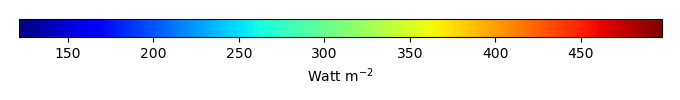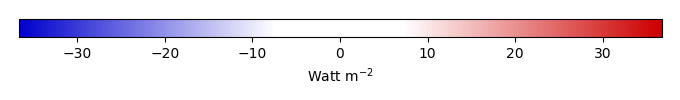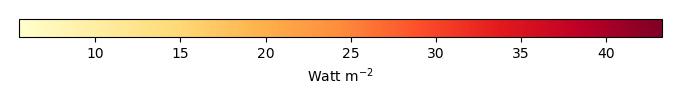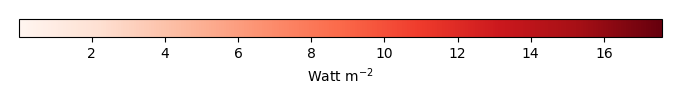# Mean State

Period Mean (original grids) [Watt m-2]
Model Period Mean (intersection) [Watt m-2]
Model Period Mean (complement) [Watt m-2]
Benchmark Period Mean (intersection) [Watt m-2]
Benchmark Period Mean (complement) [Watt m-2]
Bias [Watt m-2]
RMSE [Watt m-2]
Phase Shift [months]
Bias Score 
RMSE Score 
Seasonal Cycle Score 
Spatial Distribution Score 
Interannual Variability Score 
Overall Score 
Benchmark [-] 453.
CLM4 [-] 457. 457. 453. 460. 4.84 13.4 1.57 0.544 0.379 0.765 0.949 0.737 0.626
CLM4.5 [-] 461. 461. 453. 460. 8.90 16.0 1.54 0.439 0.375 0.767 0.965 0.729 0.608
CLM5 [-] 462. 462. 453. 460. 10.0 15.8 1.71 0.365 0.384 0.751 0.986 0.724 0.599
Period Mean (original grids) [Watt m-2]
Model Period Mean (intersection) [Watt m-2]
Model Period Mean (complement) [Watt m-2]
Benchmark Period Mean (intersection) [Watt m-2]
Benchmark Period Mean (complement) [Watt m-2]
Bias [Watt m-2]
RMSE [Watt m-2]
Phase Shift [months]
Bias Score 
RMSE Score 
Seasonal Cycle Score 
Spatial Distribution Score 
Interannual Variability Score 
Overall Score 
Benchmark [-] 471.
CLM4 [-] 478. 479. 471. 456. 6.98 20.8 0.812 0.602 0.471 0.935 0.976 0.788 0.707
CLM4.5 [-] 483. 484. 471. 456. 12.0 23.5 0.808 0.510 0.478 0.934 0.999 0.781 0.697
CLM5 [-] 485. 485. 471. 456. 13.4 23.9 0.875 0.498 0.469 0.929 1.00 0.760 0.688
Period Mean (original grids) [Watt m-2]
Model Period Mean (intersection) [Watt m-2]
Model Period Mean (complement) [Watt m-2]
Benchmark Period Mean (intersection) [Watt m-2]
Benchmark Period Mean (complement) [Watt m-2]
Bias [Watt m-2]
RMSE [Watt m-2]
Phase Shift [months]
Bias Score 
RMSE Score 
Seasonal Cycle Score 
Spatial Distribution Score 
Interannual Variability Score 
Overall Score 
Benchmark [-] 443.
CLM4 [-] 446. 446. 443. 444. 2.95 17.0 0.787 0.589 0.522 0.908 0.927 0.665 0.689
CLM4.5 [-] 451. 451. 443. 444. 8.04 19.1 0.838 0.505 0.515 0.905 0.940 0.675 0.676
CLM5 [-] 450. 449. 443. 444. 6.37 18.0 0.975 0.562 0.507 0.890 0.962 0.638 0.678
Period Mean (original grids) [Watt m-2]
Model Period Mean (intersection) [Watt m-2]
Model Period Mean (complement) [Watt m-2]
Benchmark Period Mean (intersection) [Watt m-2]
Benchmark Period Mean (complement) [Watt m-2]
Bias [Watt m-2]
RMSE [Watt m-2]
Phase Shift [months]
Bias Score 
RMSE Score 
Seasonal Cycle Score 
Spatial Distribution Score 
Interannual Variability Score 
Overall Score 
Benchmark [-] 276.
CLM4 [-] 257. 257. 275. 292. -15.2 24.6 0.0720 0.784 0.752 0.995 0.965 0.794 0.840
CLM4.5 [-] 259. 259. 275. 292. -13.8 23.1 0.0685 0.799 0.759 0.995 0.961 0.768 0.840
CLM5 [-] 265. 265. 275. 292. -7.59 19.0 0.0691 0.865 0.775 0.995 0.971 0.766 0.858
Period Mean (original grids) [Watt m-2]
Model Period Mean (intersection) [Watt m-2]
Model Period Mean (complement) [Watt m-2]
Benchmark Period Mean (intersection) [Watt m-2]
Benchmark Period Mean (complement) [Watt m-2]
Bias [Watt m-2]
RMSE [Watt m-2]
Phase Shift [months]
Bias Score 
RMSE Score 
Seasonal Cycle Score 
Spatial Distribution Score 
Interannual Variability Score 
Overall Score 
Benchmark [-] 456.
CLM4 [-] 450. 450. 453. 465. -0.720 9.44 2.02 0.360 0.368 0.686 0.986 0.667 0.572
CLM4.5 [-] 452. 452. 453. 465. 0.402 9.67 2.00 0.359 0.373 0.688 0.989 0.667 0.575
CLM5 [-] 456. 456. 453. 465. 4.81 10.5 2.03 0.307 0.384 0.682 0.986 0.671 0.569
Period Mean (original grids) [Watt m-2]
Model Period Mean (intersection) [Watt m-2]
Model Period Mean (complement) [Watt m-2]
Benchmark Period Mean (intersection) [Watt m-2]
Benchmark Period Mean (complement) [Watt m-2]
Bias [Watt m-2]
RMSE [Watt m-2]
Phase Shift [months]
Bias Score 
RMSE Score 
Seasonal Cycle Score 
Spatial Distribution Score 
Interannual Variability Score 
Overall Score 
Benchmark [-] 306.
CLM4 [-] 302. 302. 306. 329. -3.13 11.9 0.0220 0.934 0.861 0.999 0.976 0.886 0.919
CLM4.5 [-] 303. 303. 306. 329. -2.69 11.8 0.0220 0.936 0.860 0.999 0.977 0.893 0.921
CLM5 [-] 309. 309. 306. 329. 3.73 11.7 0.0258 0.920 0.867 0.998 0.976 0.890 0.920
Period Mean (original grids) [Watt m-2]
Model Period Mean (intersection) [Watt m-2]
Model Period Mean (complement) [Watt m-2]
Benchmark Period Mean (intersection) [Watt m-2]
Benchmark Period Mean (complement) [Watt m-2]
Bias [Watt m-2]
RMSE [Watt m-2]
Phase Shift [months]
Bias Score 
RMSE Score 
Seasonal Cycle Score 
Spatial Distribution Score 
Interannual Variability Score 
Overall Score 
Benchmark [-] 362.
CLM4 [-] 356. 355. 361. 395. -3.37 14.5 0.126 0.863 0.828 0.990 1.00 0.775 0.881
CLM4.5 [-] 356. 356. 361. 395. -2.66 14.3 0.114 0.869 0.828 0.992 0.999 0.770 0.881
CLM5 [-] 361. 360. 361. 395. 1.74 14.5 0.198 0.866 0.826 0.986 1.00 0.754 0.876
Period Mean (original grids) [Watt m-2]
Model Period Mean (intersection) [Watt m-2]
Model Period Mean (complement) [Watt m-2]
Benchmark Period Mean (intersection) [Watt m-2]
Benchmark Period Mean (complement) [Watt m-2]
Bias [Watt m-2]
RMSE [Watt m-2]
Phase Shift [months]
Bias Score 
RMSE Score 
Seasonal Cycle Score 
Spatial Distribution Score 
Interannual Variability Score 
Overall Score 
Benchmark [-] 450.
CLM4 [-] 451. 452. 450. 463. 4.42 18.4 0.476 0.619 0.561 0.959 0.969 0.691 0.727
CLM4.5 [-] 455. 456. 450. 463. 8.17 19.8 0.491 0.575 0.563 0.957 0.975 0.689 0.720
CLM5 [-] 457. 458. 450. 463. 10.2 20.0 0.673 0.556 0.567 0.935 0.987 0.654 0.711
Period Mean (original grids) [Watt m-2]
Model Period Mean (intersection) [Watt m-2]
Model Period Mean (complement) [Watt m-2]
Benchmark Period Mean (intersection) [Watt m-2]
Benchmark Period Mean (complement) [Watt m-2]
Bias [Watt m-2]
RMSE [Watt m-2]
Phase Shift [months]
Bias Score 
RMSE Score 
Seasonal Cycle Score 
Spatial Distribution Score 
Interannual Variability Score 
Overall Score 
Benchmark [-] 275.
CLM4 [-] 265. 265. 275. 280. -9.37 20.3 0.0384 0.855 0.751 0.997 0.967 0.873 0.866
CLM4.5 [-] 265. 265. 275. 280. -9.31 19.2 0.103 0.856 0.765 0.992 0.969 0.851 0.866
CLM5 [-] 273. 273. 275. 280. -1.59 15.1 0.0657 0.922 0.792 0.995 0.979 0.853 0.889
Period Mean (original grids) [Watt m-2]
Model Period Mean (intersection) [Watt m-2]
Model Period Mean (complement) [Watt m-2]
Benchmark Period Mean (intersection) [Watt m-2]
Benchmark Period Mean (complement) [Watt m-2]
Bias [Watt m-2]
RMSE [Watt m-2]
Phase Shift [months]
Bias Score 
RMSE Score 
Seasonal Cycle Score 
Spatial Distribution Score 
Interannual Variability Score 
Overall Score 
Benchmark [-] 448.
CLM4 [-] 447. 447. 450. 423. -4.99 14.2 0.331 0.765 0.723 0.972 1.00 0.774 0.826
CLM4.5 [-] 448. 448. 450. 423. -4.69 14.4 0.322 0.758 0.721 0.973 1.00 0.774 0.825
CLM5 [-] 450. 450. 450. 423. -2.94 14.0 0.347 0.785 0.714 0.972 0.999 0.739 0.821
Period Mean (original grids) [Watt m-2]
Model Period Mean (intersection) [Watt m-2]
Model Period Mean (complement) [Watt m-2]
Benchmark Period Mean (intersection) [Watt m-2]
Benchmark Period Mean (complement) [Watt m-2]
Bias [Watt m-2]
RMSE [Watt m-2]
Phase Shift [months]
Bias Score 
RMSE Score 
Seasonal Cycle Score 
Spatial Distribution Score 
Interannual Variability Score 
Overall Score 
Benchmark [-] 364.
CLM4 [-] 357. 357. 362. 385. -2.12 11.7 0.237 0.852 0.793 0.984 0.996 0.835 0.875
CLM4.5 [-] 356. 356. 362. 385. -2.72 10.9 0.215 0.863 0.803 0.986 0.998 0.841 0.882
CLM5 [-] 361. 361. 362. 385. 1.58 10.8 0.513 0.858 0.809 0.965 1.00 0.828 0.878
Period Mean (original grids) [Watt m-2]
Model Period Mean (intersection) [Watt m-2]
Model Period Mean (complement) [Watt m-2]
Benchmark Period Mean (intersection) [Watt m-2]
Benchmark Period Mean (complement) [Watt m-2]
Bias [Watt m-2]
RMSE [Watt m-2]
Phase Shift [months]
Bias Score 
RMSE Score 
Seasonal Cycle Score 
Spatial Distribution Score 
Interannual Variability Score 
Overall Score 
Benchmark [-] 397.
CLM4 [-] 372. 372. 376. 406. -2.67 16.3 0.474 0.724 0.666 0.948 0.999 0.721 0.787
CLM4.5 [-] 373. 373. 376. 406. -1.39 16.7 0.478 0.709 0.662 0.948 0.999 0.719 0.783
CLM5 [-] 377. 377. 376. 406. 1.88 16.1 0.535 0.713 0.669 0.943 0.999 0.704 0.783
Period Mean (original grids) [Watt m-2]
Model Period Mean (intersection) [Watt m-2]
Model Period Mean (complement) [Watt m-2]
Benchmark Period Mean (intersection) [Watt m-2]
Benchmark Period Mean (complement) [Watt m-2]
Bias [Watt m-2]
RMSE [Watt m-2]
Phase Shift [months]
Bias Score 
RMSE Score 
Seasonal Cycle Score 
Spatial Distribution Score 
Interannual Variability Score 
Overall Score 
Benchmark [-] 464.
CLM4 [-] 452. 452. 465. 441. -12.5 21.9 0.404 0.732 0.747 0.968 0.998 0.663 0.809
CLM4.5 [-] 452. 452. 465. 441. -12.5 21.9 0.405 0.733 0.745 0.968 0.997 0.659 0.808
CLM5 [-] 454. 454. 465. 441. -11.1 21.4 0.446 0.748 0.740 0.965 0.995 0.649 0.806
Period Mean (original grids) [Watt m-2]
Model Period Mean (intersection) [Watt m-2]
Model Period Mean (complement) [Watt m-2]
Benchmark Period Mean (intersection) [Watt m-2]
Benchmark Period Mean (complement) [Watt m-2]
Bias [Watt m-2]
RMSE [Watt m-2]
Phase Shift [months]
Bias Score 
RMSE Score 
Seasonal Cycle Score 
Spatial Distribution Score 
Interannual Variability Score 
Overall Score 
Benchmark [-] 278.
CLM4 [-] 270. 270. 277. 289. -8.21 17.8 0.0696 0.851 0.778 0.995 0.961 0.786 0.858
CLM4.5 [-] 270. 270. 277. 289. -7.99 17.1 0.0688 0.853 0.789 0.995 0.961 0.776 0.860
CLM5 [-] 277. 276. 277. 289. -1.61 14.2 0.0692 0.897 0.807 0.995 0.966 0.779 0.875
Period Mean (original grids) [Watt m-2]
Model Period Mean (intersection) [Watt m-2]
Model Period Mean (complement) [Watt m-2]
Benchmark Period Mean (intersection) [Watt m-2]
Benchmark Period Mean (complement) [Watt m-2]
Bias [Watt m-2]
RMSE [Watt m-2]
Phase Shift [months]
Bias Score 
RMSE Score 
Seasonal Cycle Score 
Spatial Distribution Score 
Interannual Variability Score 
Overall Score 
Benchmark [-] 444.
CLM4 [-] 444. 444. 442. 455. 6.79 17.1 0.558 0.508 0.560 0.942 0.837 0.762 0.695
CLM4.5 [-] 443. 443. 442. 455. 4.68 15.1 0.543 0.568 0.567 0.941 0.916 0.753 0.718
CLM5 [-] 445. 445. 442. 455. 6.86 15.5 0.625 0.530 0.578 0.938 0.931 0.735 0.715
Period Mean (original grids) [Watt m-2]
Model Period Mean (intersection) [Watt m-2]
Model Period Mean (complement) [Watt m-2]
Benchmark Period Mean (intersection) [Watt m-2]
Benchmark Period Mean (complement) [Watt m-2]
Bias [Watt m-2]
RMSE [Watt m-2]
Phase Shift [months]
Bias Score 
RMSE Score 
Seasonal Cycle Score 
Spatial Distribution Score 
Interannual Variability Score 
Overall Score 
Benchmark [-] 386.
CLM4 [-] 380. 380. 384. 429. -1.49 9.00 0.148 0.903 0.847 0.990 1.00 0.893 0.913
CLM4.5 [-] 381. 381. 384. 429. -0.369 9.36 0.145 0.899 0.841 0.989 1.00 0.895 0.911
CLM5 [-] 387. 387. 384. 429. 5.60 10.2 0.275 0.867 0.850 0.981 0.999 0.892 0.907
Period Mean (original grids) [Watt m-2]
Model Period Mean (intersection) [Watt m-2]
Model Period Mean (complement) [Watt m-2]
Benchmark Period Mean (intersection) [Watt m-2]
Benchmark Period Mean (complement) [Watt m-2]
Bias [Watt m-2]
RMSE [Watt m-2]
Phase Shift [months]
Bias Score 
RMSE Score 
Seasonal Cycle Score 
Spatial Distribution Score 
Interannual Variability Score 
Overall Score 
Benchmark [-] 319.
CLM4 [-] 314. 315. 318. 343. -2.51 11.1 0.0839 0.909 0.840 0.994 1.00 0.851 0.906
CLM4.5 [-] 315. 315. 318. 343. -1.73 11.2 0.0960 0.908 0.837 0.994 0.998 0.842 0.903
CLM5 [-] 322. 322. 318. 343. 4.63 11.7 0.170 0.894 0.841 0.989 0.999 0.841 0.901
Period Mean (original grids) [Watt m-2]
Model Period Mean (intersection) [Watt m-2]
Model Period Mean (complement) [Watt m-2]
Benchmark Period Mean (intersection) [Watt m-2]
Benchmark Period Mean (complement) [Watt m-2]
Bias [Watt m-2]
RMSE [Watt m-2]
Phase Shift [months]
Bias Score 
RMSE Score 
Seasonal Cycle Score 
Spatial Distribution Score 
Interannual Variability Score 
Overall Score 
Benchmark [-] 376.
CLM4 [-] 371. 373. 376. 379. -3.14 14.6 0.109 0.859 0.819 0.993 0.999 0.810 0.883
CLM4.5 [-] 372. 373. 376. 379. -2.51 13.9 0.0950 0.862 0.827 0.994 1.00 0.813 0.887
CLM5 [-] 377. 378. 376. 379. 2.07 14.8 0.131 0.862 0.814 0.991 0.999 0.770 0.875
Period Mean (original grids) [Watt m-2]
Model Period Mean (intersection) [Watt m-2]
Model Period Mean (complement) [Watt m-2]
Benchmark Period Mean (intersection) [Watt m-2]
Benchmark Period Mean (complement) [Watt m-2]
Bias [Watt m-2]
RMSE [Watt m-2]
Phase Shift [months]
Bias Score 
RMSE Score 
Seasonal Cycle Score 
Spatial Distribution Score 
Interannual Variability Score 
Overall Score 
Benchmark [-] 449.
CLM4 [-] 451. 451. 449. 458. 2.92 12.8 1.19 0.522 0.396 0.827 0.765 0.705 0.602
CLM4.5 [-] 454. 454. 449. 458. 5.38 13.7 1.15 0.463 0.395 0.836 0.771 0.704 0.594
CLM5 [-] 455. 455. 449. 458. 6.39 13.2 1.15 0.442 0.404 0.834 0.863 0.685 0.605
Period Mean (original grids) [Watt m-2]
Model Period Mean (intersection) [Watt m-2]
Model Period Mean (complement) [Watt m-2]
Benchmark Period Mean (intersection) [Watt m-2]
Benchmark Period Mean (complement) [Watt m-2]
Bias [Watt m-2]
RMSE [Watt m-2]
Phase Shift [months]
Bias Score 
RMSE Score 
Seasonal Cycle Score 
Spatial Distribution Score 
Interannual Variability Score 
Overall Score 
Benchmark [-] 440.
CLM4 [-] 443. 442. 440. 430. 1.63 13.7 0.721 0.649 0.596 0.908 0.944 0.809 0.750
CLM4.5 [-] 446. 445. 440. 430. 4.54 14.4 0.841 0.615 0.589 0.895 0.930 0.812 0.738
CLM5 [-] 447. 447. 440. 430. 6.01 12.7 0.806 0.675 0.603 0.899 0.981 0.774 0.756
Period Mean (original grids) [Watt m-2]
Model Period Mean (intersection) [Watt m-2]
Model Period Mean (complement) [Watt m-2]
Benchmark Period Mean (intersection) [Watt m-2]
Benchmark Period Mean (complement) [Watt m-2]
Bias [Watt m-2]
RMSE [Watt m-2]
Phase Shift [months]
Bias Score 
RMSE Score 
Seasonal Cycle Score 
Spatial Distribution Score 
Interannual Variability Score 
Overall Score 
Benchmark [-] 387.
CLM4 [-] 379. 378. 386. 394. -6.80 19.5 0.594 0.602 0.615 0.937 0.996 0.734 0.750
CLM4.5 [-] 381. 380. 386. 394. -4.82 19.0 0.576 0.615 0.613 0.937 0.997 0.744 0.753
CLM5 [-] 383. 382. 386. 394. -2.95 18.3 0.590 0.613 0.627 0.936 0.999 0.718 0.753

# Temporally integrated period mean

BENCHMARK MEANMODEL MEANBIASBIAS SCORERMSERMSE SCOREBENCHMARK INTERANNUAL VARIABILITYMODEL INTERANNUAL VARIABILITYINTERANNUAL VARIABILITY SCOREBENCHMARK MAX MONTHMODEL MAX MONTHDIFFERENCE IN MAX MONTHSEASONAL CYCLE SCORESPATIAL TAYLOR DIAGRAMMODEL COLORS# Spatially integrated regional mean

MODEL COLORSREGIONAL MEANANNUAL CYCLEMONTHLY ANOMALYANNUAL CYCLE# All Models

BenchmarkCLM4CLM4.5CLM5# Data Information

creation_date: Mon Jun 30 23:35:20 PDT 2014

source_file: This product is generated from monthly 1 degree GEWEX SRB Radiation observations